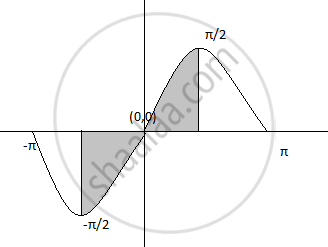HSC Science (General) 12th Board ExamMaharashtra State Board
Share

# Find the area of the region bounded by the curve y = sinx, the lines x=-π/2 , x=π/2 and X-axis - HSC Science (General) 12th Board Exam - Mathematics and Statistics

ConceptArea of the Region Bounded by a Curve and a Line

#### Question

Find the area of the region bounded by the curve y = sinx, the lines x=-π/2 , x=π/2 and X-axis

#### Solution

Required area=int_(-pi/2)^(pi/2)y dx , where y=sinx=2int_(-pi/2)^(pi) sinx dx

=2[cosx]_(-pi/2)^(pi)

=2[1-0]

=2 sq.unit

Is there an error in this question or solution?

#### APPEARS IN

2013-2014 (October) (with solutions)
Question 4.2.5 | 2.00 marks

#### Video TutorialsVIEW ALL 

Solution Find the area of the region bounded by the curve y = sinx, the lines x=-π/2 , x=π/2 and X-axis Concept: Area of the Region Bounded by a Curve and a Line.
S